# Related Videos

## Simplify $3+5+7$

Go!
1
2
3
4
5
6
7
8
9
0
a
b
c
d
f
g
m
n
u
v
w
x
y
z
.
(◻)
+
-
×
◻/◻
/
÷
2

e
π
ln
log
log
lim
d/dx
Dx
|◻|
=
>
<
>=
<=
sin
cos
tan
cot
sec
csc

asin
acos
atan
acot
asec
acsc

sinh
cosh
tanh
coth
sech
csch

asinh
acosh
atanh
acoth
asech
acsch

### Videos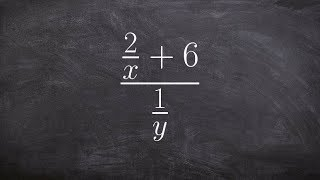### Algebra 2 - Math tutorial for simplifying complex fractions, ((2/x) + 6) / (1/y)

https://www.youtube.com/watch?v=iprRyYew3hU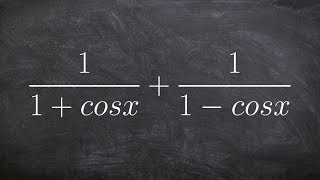### Adding and simplifying two trigonometric fractions

https://www.youtube.com/watch?v=1PX3FYF8vHg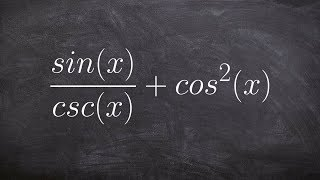### Tutorial - Simplify a trigonometric expression and then find the sum ex 31, (sinx/cscx) + (cosx)^2

https://www.youtube.com/watch?v=gim3YN7vOw8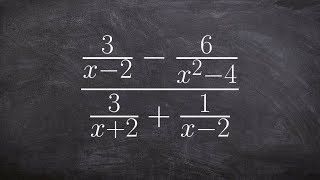### Tutorial - Simplifying complex fractions ex 38, ((3/(x-2)) - (6/(x^2-4)) / 3/(X+2) + 1/(X-2)

https://www.youtube.com/watch?v=4vzjCLBe4V0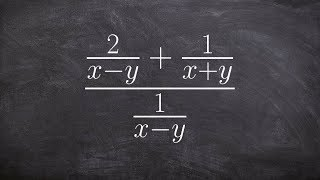### Algebra 2 - dividing two rational expressions by combining expressions [(2/x-y) + (1/x+y)] / (1/x-y)

https://www.youtube.com/watch?v=4F99_065vj4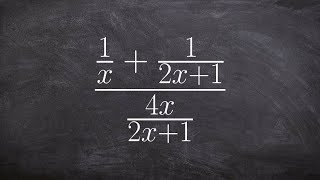### Tutorial - Simplifying a complex rational expression, ((1/x) + (1/(2x+1))) / (4x/(2x+1))

https://www.youtube.com/watch?v=1ZaczA77hS8
$3+5+7$

### Main topic:

Addition of numbers

~ 0.01 s